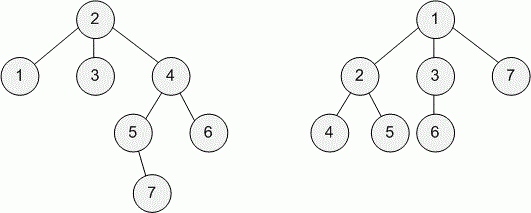Competitions

# Tree with small distances

You are given an undirected tree consisting of n vertices. An undirected tree is a connected undirected graph with n1 edges.

Your task is to add the minimum number of edges in such a way that the length of the shortest path from the vertex 1 to any other vertex is at most 2. Note that you are not allowed to add loops and multiple edges.

#### Input

The first line contains one integer n (2n2 * 105) — the number of vertices in the tree.

The following n1 lines contain edges: edge i is given as a pair of vertices ui, vi (1ui, vin). It is guaranteed that the given edges form a tree. It is guaranteed that there are no loops and multiple edges in the given edges.

#### Output

Print a single integer — the minimum number of edges you have to add in order to make the shortest distance from the vertex 1 to any other vertex at most 2. Note that you are not allowed to add loops and multiple edges.Time limit 1 second
Memory limit 128 MiB
Input example #1
7
1 2
2 3
2 4
4 5
4 6
5 7

Output example #1
2

Input example #2
7
1 2
1 3
2 4
2 5
3 6
1 7

Output example #2
0